• 引言 实际应用中，我们也会经常使用到直线轨迹，然而（5）中讲的三次多项式并不能满足我们的直线轨迹。然而如果单纯地使用直线...直线轨迹规划 多段直线轨迹规划 符号的设定与相关公式 公式 影响加速度的因素 补充...


引言
实际应用中，我们也会经常使用到直线轨迹，然而（5）中讲的三次多项式并不能满足我们的直线轨迹。然而如果单纯地使用直线轨迹，线段间的转折点会让速度不连续，如何又能使用直线轨迹又能满足速度连续呢？在这里引出一次多项式（直线）与二次多项式的结合使用。
目录
直线轨迹规划
多段直线轨迹规划
符号的设定与相关公式
公式
影响加速度的因素
补充说明
相关例题

直线轨迹规划
一般使用一次多项式（即直线方程式）与二次多项式一起使用，二次多项式的目的是为整个线段提供等加速与等减速，由此达到速度连续无断层的目的。
其实之前也讲过，轨迹规划核心就是拟合线嘛。
三次多项式需要参数  ，因此在三次多项式的轨迹规划中，求解是核心。
所以我认为直线轨迹规划的核心有两个：
一个是要知道速度 ——求解直线段的关键参数。一个是要知道加速度 ——求解曲线段的关键参数。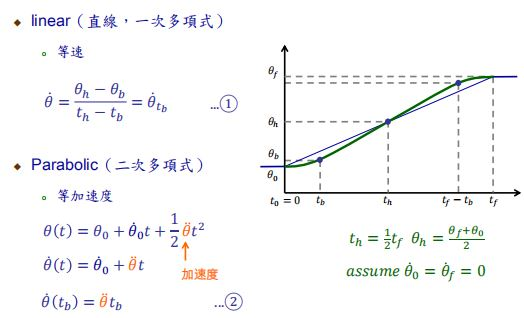统一符号
整个线段时刻区间为 [  ,  ] 。
其中 和以前一样是角度位置，[点]代表速度，即对求导。[点点]代表加速度，即对[点]求导。
衔接了一次多项式和二次多项式，为直线轨迹的起点对应时刻，可作为“中间点”看待。
为直线轨迹的终点对应时刻，可作为“中间点”看待。
为直线轨迹经历时间的一半，也是整个线段的一半。

由图中的等式，我们和之前一样，当达到中间点的时刻  需要将中间点前后的式子带入并使之相等，就能得到连续的速度。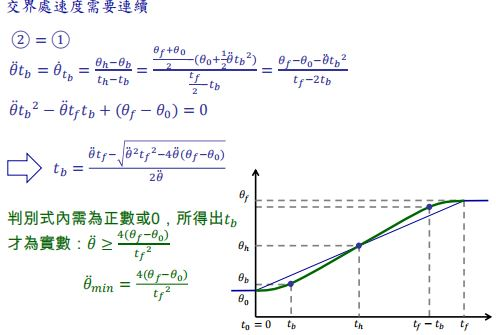纯直线轨迹规划、直线轨迹规划、三次多项式轨迹规划 拟合直线轨迹的区别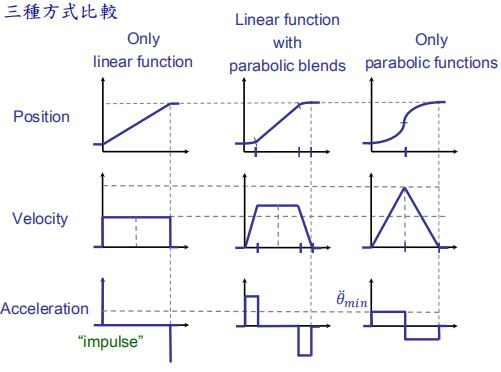或许就有人纳闷了，使用直线轨迹规划，第二列的加速度也“不连续”啊，其实真实上电控手臂的加速度变化会通过电产生一个扭力达到变化，其实电压也是一个从零慢慢变化的过程，但是电比机械响应得快，所以这样的加速度的需求是可以满足的。

上面那个例子是为了更好地说明举出的特殊例子——头尾两端速度都是0。实际上一般我们在做轨迹规划时常采用的是多段的直曲结合表示直线的轨迹规划，而多段中的许多中间段的头尾速度都不为0，那怎么做呢？看下面。
多段直线轨迹规划

符号的设定与相关公式

统一符号（1）中间多段的符号设定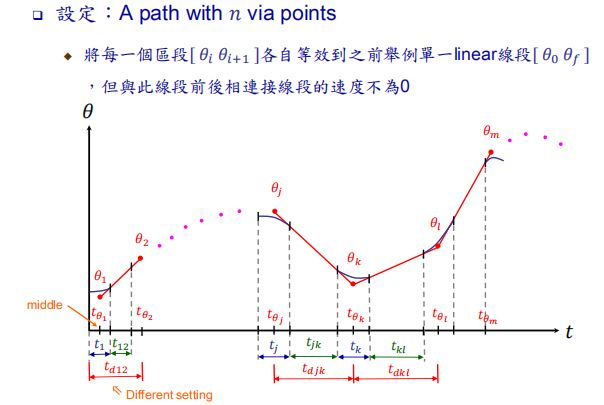是中间点，与中间点中夹着“半个曲线（属于的二次多项式）+一整个直线+半个曲线”（属于的二次多项式）。
代表中间点  的二次多项式段（曲线段）的时间
代表中间点 到  的直线段的时间
代表中间点 到  （包括曲线和直线）的时间差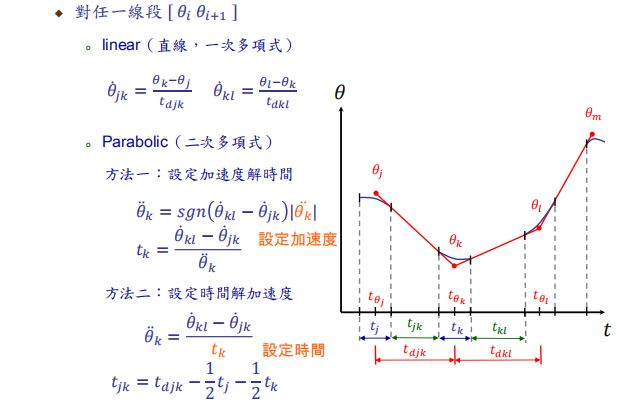其中sgn()的作用是确定方向，正负号。这些公式都是初高中的物理学学过的了....不多说。

统一符号（2）头尾段的符号设定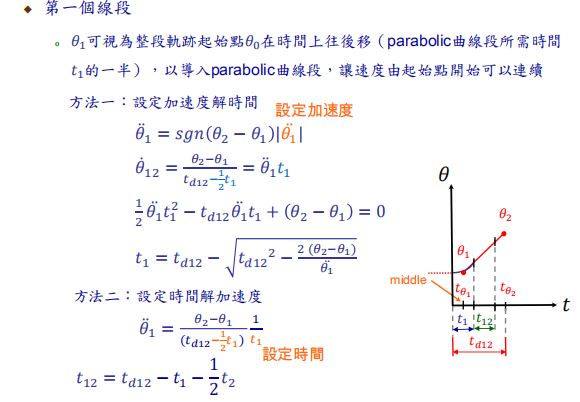【头段】将起点后移一段时间（一般是曲线段时间的一半），前面导入一段圆滑的曲线连接。起点就会到达的新的位置（即图中所示点）。此时就能将“起点”当作“中间点”了，更好地方便运算。定义完以后就能列出物理等式了，如上图。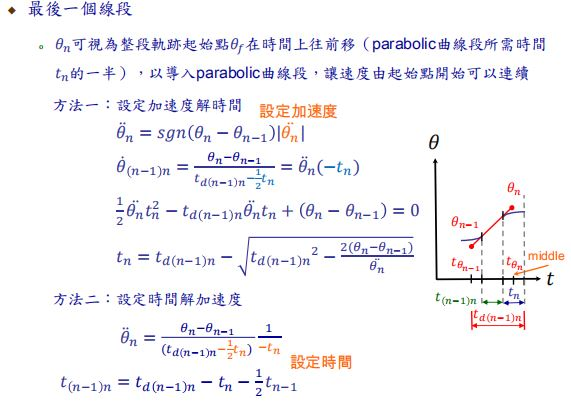【尾段】将终点前移一段时间，后面导入一段圆滑的曲线连接。终就会到达的新的位置（即图中所示点）。此时就能将“终点”当作“中间点”了，更好地方便运算。定义完以后列出物理等式，如上图。

公式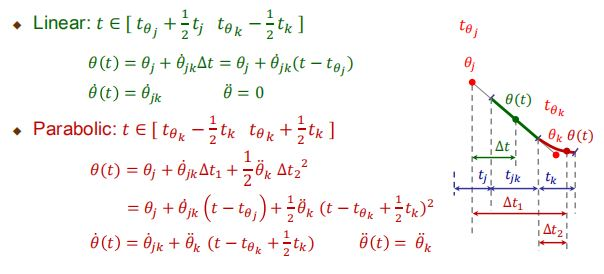影响加速度的因素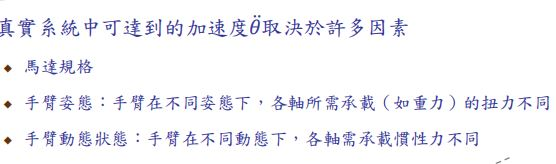这个涉及到了动力学，稍微了解下就行。

补充说明

（1） 规划完后，会发现原来我们设定的中间点，都到达不了怎么办？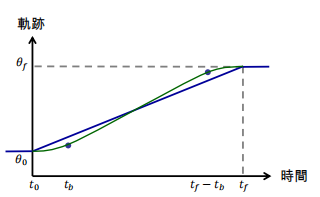在必须通过的中间点附近添加一些假的中间点，这样我们设定的中间点会落在直线段上，且不管二次式轨迹如何规划都影响不到我们直线的轨迹。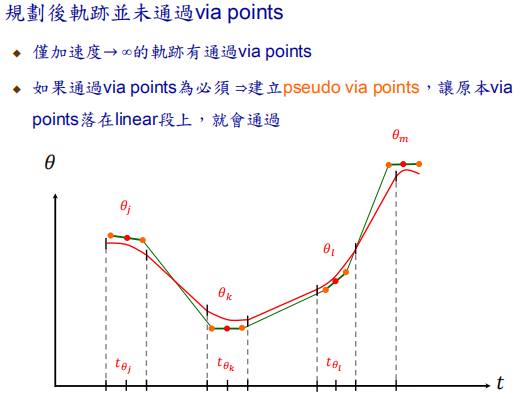（2）为什么常在Cartesian space下做直线规划？
除了更直观以外。如果我们在Joint space下做“等速段”的直线轨迹运动，想想好像是可行的，等角速嘛。但是实际上Joint space和Actuator space息息相关，表面上Joint space是等角速，其实实际上我们还要考虑它关节内部的速度等等，麻烦。

相关例题
仍然使用以前的例子，使用直线轨迹规划求解。（Cartesian下解题）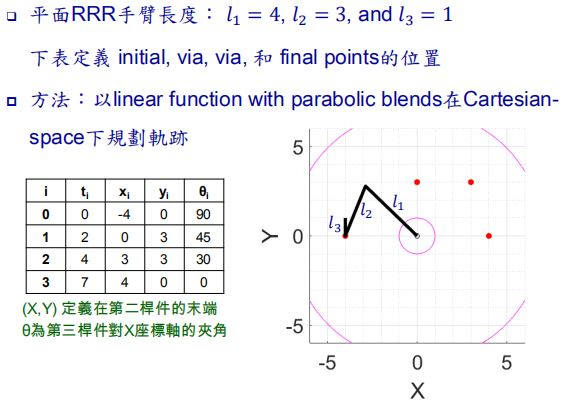解析题目，题目给出了4个坐标点的三个关键量  、 、 ， 要求在Cartesian下用直线轨迹规划方法解题，也就是要求我们分别求出这三个关键量的连续的直线轨迹。
与之前三次多项式轨迹规划不同的是，本章直线轨迹规划（曲+直）在意的是加速度、速度公式，有了加速度的物理公式，才能规划出曲线部分在图上的表达，有了速度的物理公式，才能规划出直线在图上的表达。所以第一步：
（1）连接需求（题目）规定要到达的点，知道大概的轨迹图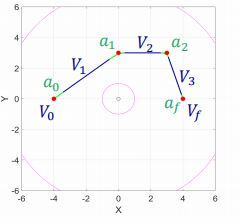其中蓝色速度代表直线部分，绿色加速度代表曲线部分，红色为需要到的点。
可以看到，蓝色线段与绿色线段一共有7段。
（2）先求出需求点和每段的速度、再求出加速度
注：每段加速段的时长为0.5秒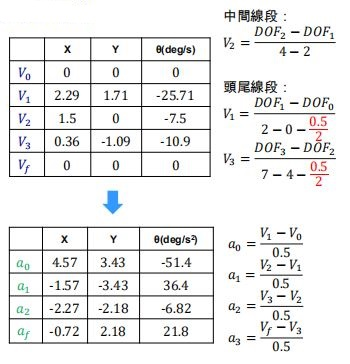上文【统一符号】中已经定义过，头尾点需要内移了加速段的一半时间，所以时长如红色部分所示要减去（0.5 / 2）
（3）使用求出的速度和加速度，构建轨迹的连续方程式。
讲直线轨迹规划的时候已经给过公式了: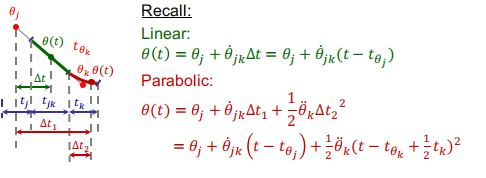结合公式，我们就能求出题目要求的  轴的七段方程式：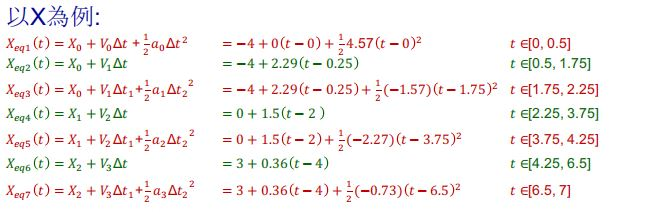轴的轨迹方程式已经求出，剩下两个 、 也是类似的求法，在此不列出。
最终得到的三条轨迹就是：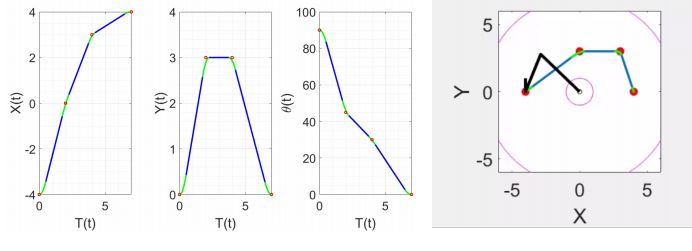（4）检验可行性
求出答案后最好IK逆向算出驱动转角，再FK顺向得到移动位置，看看该轨迹在现实中是否可行。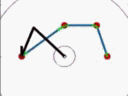感谢：课程内容和PPT来自台湾林沛群教授的《机器人学》！


展开全文matlab
• 用Robotics Toolbox for MATLAB做的一个Motoman机器人的关节空间轨迹规划和笛卡尔空间轨迹规划代码
• 从这个角度理解，轨迹规划就是一种路径规划，当路径规划过程要满足无人车辆的纵向和横向动力学约束时，就成为轨迹轨迹规划。路径规划和轨迹规划既可以在状态空间中表示，也可以在笛卡尔坐标系中表示。
        对于无人车辆来说，全局路径点只要包含空间位置信息即可，也可以包含姿态信息，而不需要与时间相关，但局部规划时，则可以考虑时间信息。这里规定轨迹点也是一种路径点，即当路径点信息中加入时间约束，就可以被称为轨迹点。从这个角度理解，轨迹规划就是一种路径规划，当路径规划过程要满足无人车辆的纵向和横向动力学约束时，就成为轨迹轨迹规划。路径规划和轨迹规划既可以在状态空间中表示，也可以在笛卡尔坐标系中表示。

路径跟踪过程中，参考路径曲线可与时间参数无关，跟踪控制时，可以假设无人车辆以当前速度匀速前进，以一定的代价规则使行驶路径趋近于参考路径；而轨迹跟踪时，参考路径曲线与时间和空间均相关，并要求无人车辆在规定的时间内到达某一预设好的参考路径点。

路径跟踪不同于轨迹跟踪，不受制于时间约束，只需要在一定误差范围内跟踪参考路径。路径跟踪中的运动控制就是寻找一个有界的控制输入序列，以使无人车辆从一个初始位形到设定的期望位形。

参考：《无人驾驶车辆模型预测控制》，北京理工大学出版社

有一个非常全的，python写的，路径规划/运动规则/轨迹规划的仓库，见：https://atsushisakai.github.io/PythonRobotics/#what-is-this

展开全文路径
• 一、 无人车的运动规划和轨迹规划 路径规划(motion planning)：不考虑临时或者移动障碍物的前提下进行的规划；轨迹规划(trajectories planning)：考虑实际临时或者移动障碍物，考虑速度，动力学约束的情况下，尽量...
一、 无人车的运动规划和轨迹规划

路径规划(motion planning)：不考虑临时或者移动障碍物的前提下进行的规划；轨迹规划(trajectories planning)：考虑实际临时或者移动障碍物，考虑速度，动力学约束的情况下，尽量按照规划路径进行轨迹规划。如果没有障碍物，A,B两点间的运动规划和轨迹规划是一个概念。

二、机械臂的轨迹规划

首先，机械臂的轨迹规划必须考虑空间障碍物的存在。

1. 正向运动学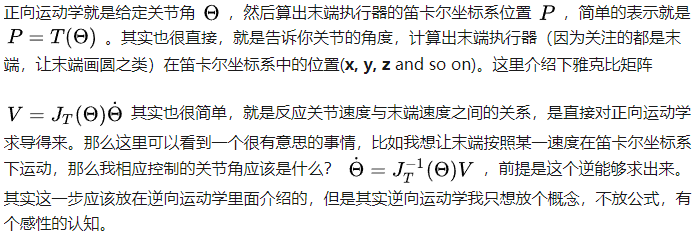2. 逆向运动学灵巧工作空间：机械臂末端执行器可以从各个方向到达的空间区域。

可达工作区间：机械臂末端执行器至少从一个方向上有一个方位可以到达的区域。

6自由度机械臂末端位置逆运动学的解：一个末端位置的解的最大数和等于零的连杆长度参数有关，非零连杆长度数目越多，解的个数越多，对于6R机械臂，最多可达到16个。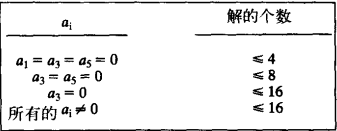6自由度机械臂，计算数值解比计算解析解（封闭解）耗时，所以现在机械臂设计都让其含有解析解，以简化求解过程。存在解析解机械臂的充分条件：三个相邻的关节轴线相较于一点。当今6自由度机械臂，几乎都有三根相交轴。

3. 对于多关节机械臂的轨迹规划问题，已知末端位置变化，通过雅各比矩阵运用逆运动学求出个关节角相应的变化。

4. 机械臂的轨迹是指：每个自由度的位置，速度和加速度的时间历程。

最理想的状态是给定工具坐标系和工作台坐标系，由计算机规划出一条路径。但实际过程中要加入一些列的中间点（其实叫做中间坐标系，表示了工具的位置和姿态，作为过渡点，），以及考虑各中间点的时间间隔。

4.1 关节空间的规划方法

4.1.1利用多项式法：通过静运动学变换矩阵和终止时刻末端位置可以计算出各关节角度，然后再利用多项式轨迹规划的方法，规划出一条期望轨迹，且各关节之间期望轨迹互相独立，同时进行。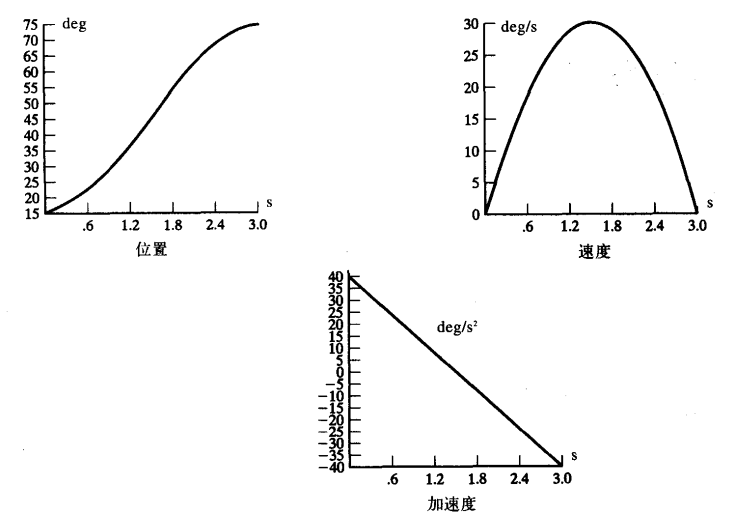图：三次多项式轨迹规划特点，除了初始，终止时刻，速度均不为零，速度为抛物线，加速度为直线。

单目标点时只用一次轨迹规划就可以，但实际情况会有插入若干个中间点，类似于单目标点轨迹规划，每个中间点看做一个末端位置，使用若干次多项式轨迹规划方法，但此时末端位置（中间点）的速度不为零。

4.1.2 与抛物线拟合的线性函数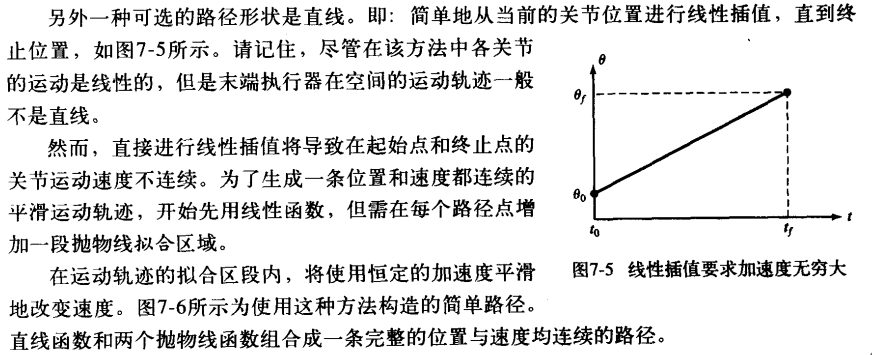这种方法，也可以插入若干中间点。

4.2笛卡尔空间规划方法

4.3路径的实时生成

首先，路径规划和路径生成的区别：路径规划是静止状态下利用多项式轨迹规划的方法规划出来的虚拟路径，是一组数据；而路径生成，是路径生成器利用这些数据实时更新theta，dot_theta，ddot_theta，生成路径。

三、无人车轨迹规划与机械臂轨迹规划的区别

无人车轨迹规划是把车看成一个质点，在笛卡尔空间下的规划；机械臂的轨迹规划要考虑每个关节电机的变化，在关节空间下进行规划。但两者在轨迹上都是运动学的规划，实际运行时需要考虑动力学的约束。

四、机械臂轨迹规划的学习指南


展开全文• 1. 轨迹规划是什么？在机器人导航过程中，如何控制机器人从A点移动到B点，通常称之为运动规划。运动规划一般又分为两步： 路径规划：在地图（栅格地图、四\八叉树、RRT地图等）中搜索一条从A点到B点的路径，由一系列...
1. 轨迹规划是什么？

在机器人导航过程中，如何控制机器人从A点移动到B点，通常称之为运动规划。运动规划一般又分为两步：

路径规划：在地图（栅格地图、四\八叉树、RRT地图等）中搜索一条从A点到B点的路径，由一系列离散的空间点（waypoint）组成。
轨迹规划：由于路径点可能比较稀疏、而且不平滑，为了能更好的控制机器人运动，需要将稀疏的路径点变成平滑的曲线或稠密的轨迹点，也就是轨迹。

2. 轨迹是什么？

轨迹一般用n阶多项式(polynomial)来表示，即
p(t)=p0+p1t+p2t2...+pntn=∑i=0npitip(t) = p_0+p_1t+p_2t^2...+p_nt^n=\sum_{i=0}^{n}p_it^i
其中p0,p1,...,pn$p_0,p_1,...,p_n$为轨迹参数（n+1个），设参数向量p=[p0,p1,...,pn]T$p=[p_0,p_1,...,p_n]^T$，则轨迹可以写成向量形式，
p(t)=[1,t,t2,...,tn]⋅pp(t) = [1,t,t^2,...,t^n]\cdot p
对于任意时刻t$t$，可以根据参数计算出轨迹的位置P(osition)，速度V(elocity)，加速度A(cceleration)，jerk，snap等。
v(t)=p′(t)=[0,1,2t,3t2,4t3,...,ntn−1]⋅pa(t)=p′′(t)=[0,0,2,6t,12t2,...,n(n−1)tn−2]⋅pjerk(t)=p(3)(t)=[0,0,0,6,24t,...,n!(n−3!)tn−3]⋅psnap(t)=p(4)(t)=[0,0,0,0,24,...,n!(n−4!)tn−4]⋅p\begin{equation}
v(t) = p'(t) = [0,1,2t,3t^2,4t^3,...,nt^{n-1}]\cdot p\\
a(t) = p''(t)=[0,0,2,6t,12t^2,...,n(n-1)t^{n-2}]\cdot p\\
jerk(t)=p^{(3)}(t) =[0,0,0,6,24t,...,\frac{n!}{(n-3!)}t^{n-3}]\cdot p \\
snap(t)=p^{(4)}(t) =[0,0,0,0,24,...,\frac{n!}{(n-4!)}t^{n-4}]\cdot p
\end{equation}

一个多项式曲线过于简单，一段复杂的轨迹很难用一个多项式表示，所以将轨迹按时间分成多段，每段各用一条多项式曲线表示，形如：
p(t)=⎧⎩⎨⎪⎪⎪⎪⎪⎪[1,t,t2,...,tn]⋅p1   t0≤t<t1[1,t,t2,...,tn]⋅p2   t1≤t<t2...[1,t,t2,...,tn]⋅pk   tk−1≤t<tk\begin{equation}
p(t) =
\begin{cases}
[1,t,t^2,...,t^n]\cdot p_1~~~t_0\leq t<t_1\\
[1,t,t^2,...,t^n]\cdot p_2~~~t_1\leq t<t_2\\
...\\
[1,t,t^2,...,t^n]\cdot p_k~~~t_{k-1}\leq t<t_k\\
\end{cases}
\end{equation}
k$k$为轨迹的段数，pi=[pi0,pi1,...,pin]T$p_i=[p_{i_0},p_{i_1},...,p_{i_n}]^T$为第i段轨迹的参数向量。

此外，实际问题中的轨迹往往是二维、三维甚至更高维，通常每个维度单独求解轨迹。

3. Minimum Snap轨迹规划

轨迹规划的目的：求轨迹的多项式参数p1,...,pk$p_1,...,p_k$。
我们可能希望轨迹满足一系列的约束条件，比如：希望设定起点和终点的位置、速度或加速度，希望相邻轨迹连接处平滑（位置连续、速度连续等），希望轨迹经过某些路径点，设定最大速度、最大加速度等，甚至是希望轨迹在规定空间内（corridor）等等。
通常满足约束条件的轨迹有无数条，而实际问题中，往往需要一条特定的轨迹，所以又需要构建一个最优的函数，在可行的轨迹中找出“最优”的那条特定的轨迹。
所以，我们将问题建模（fomulate）成一个约束优化问题，形如：
minf(p)s.t.  Aeqp=beq,        Aieqp≤bieq\begin{equation}
\min f(p)\\
s.t.~~A_{eq}p = b_{eq},\\
~~~~~~~~A_{ieq}p \leq b_{ieq}
\end{equation}
这样，就可以通过最优化的方法求解出目标轨迹参数p$p$。注意：这里的轨迹参数p$p$是多端polynomial组成的大参数向量p=[pT1,pT2,...,pTk]T$p = [p_1^T,p_2^T,...,p_k^T]^T$。
我们要做的就是：将优化问题中的f(p)$f(p)$函数和Aeq,beq,Aieq,bieq$A_{eq},b_{eq},A_{ieq},b_{ieq}$参数给列出来，然后丢到优化器中求解轨迹参数p。
Minimum Snap顾名思义，Minimum Snap中的最小化目标函数是Snap（加加加速度），当然你也可以最小化Acceleration（加速度）或者Jerk（加加速度），至于它们之间有什么区别，quora上有讨论。一般不会最小化速度。
minimum snap: minf(p)=min(p(4)(t))2minimum jerk: minf(p)=min(p(3)(t))2minimum acce: minf(p)=min(p(2)(t))2\begin{equation}
minimum~snap:~\min f(p)=\min (p^{(4)}(t))^2 \\
minimum~jerk:~\min f(p)=\min (p^{(3)}(t))^2 \\
minimum~acce:~\min f(p)=\min (p^{(2)}(t))^2 \\
\end{equation}

4. 一个简单的例子

给定包含起点终点在内的k+1个二维路径点pt0,pt1,...,ptk，pti=(xi,yi)$pt_0,pt_1,...,pt_k，pt_i=(x_i,y_i)$，给定起始速度和加速度为v0,a0$v_0,a_0$，末端加速度为ve,ae$v_e,a_e$，给定时间T，规划出经过所有路径点的平滑轨迹。

a. 初始轨迹分段与时间分配

根据路径点，将轨迹分为k段，计算每段的距离，按距离平分时间T(匀速时间分配)，得到时间序列t0,t1,...,tk$t_0,t_1,...,t_k$。对x,y维度单独规划轨迹。后面只讨论一个维度。
时间分配的方法：匀速分配或梯形分配，假设每段polynomial内速度满足匀速或梯形速度变化，根据每段的距离将总时间T分配到每段。
这里的轨迹分段和时间分配都是初始分配，在迭代算法中，如果corridor check和feasibility check不满足条件，会插点或增大某一段的时间，这个后续细说。

b. 构建优化函数

Minimum Snap的优化函数为：
min∫T0(p(4)(t))2dt=min∑i=1k∫titi−1(p(4)(t))2dt=min∑i=1k∫titi−1([0,0,0,0,24,...,n!(n−4!)tn−4]⋅p)T[0,0,0,0,24,...,n!(n−4!)tn−4]⋅p dt=min∑i=1kpT∫titi−1[0,0,0,0,24,...,n!(n−4!)tn−4]T[0,0,0,0,24,...,n!(n−4!)tn−4] dt p=min∑i=1kpTQip\begin{equation}
\min  \int _0^T(p^{(4)}(t))^2 {\rm d}t\\
=\min \sum_{i=1}^k \int _{t_{i-1}}^{t_i}(p^{(4)}(t))^2 {\rm d}t\\
=\min \sum_{i=1}^k \int _{t_{i-1}}^{t_i}   ([0,0,0,0,24,...,\frac{n!}{(n-4!)}t^{n-4}]\cdot p)^T[0,0,0,0,24,...,\frac{n!}{(n-4!)}t^{n-4}]\cdot p~{\rm d}t\\
=\min \sum_{i=1}^k p^T\int _{t_{i-1}}^{t_i}[0,0,0,0,24,...,\frac{n!}{(n-4!)}t^{n-4}]^T[0,0,0,0,24,...,\frac{n!}{(n-4!)}t^{n-4}]~{\rm d}t~p\\
=\min \sum_{i=1}^k p^TQ_ip
\end{equation}
其中，
Qi=∫titi−1[0,0,0,0,24,...,n!(n−4!)tn−4]T[0,0,0,0,24,...,n!(n−4!)tn−4] dt=⎡⎣⎢04×40(n−3)×404×(n−3)r!(r−4)!c!(c−4)!1(r−4)+(c−4)+1(t(r+c−7)i−t(r+c−7)i−1)⎤⎦⎥\begin{equation}
Q_i = \int _{t_{i-1}}^{t_i}[0,0,0,0,24,...,\frac{n!}{(n-4!)}t^{n-4}]^T[0,0,0,0,24,...,\frac{n!}{(n-4!)}t^{n-4}]~{\rm d}t \\
=\left[\begin{matrix}
0_{4\times 4} & 0_{4\times (n-3)}\\
0_{(n-3) \times 4} & \frac{r!}{(r-4)!}\frac{c!}{(c-4)!}\frac{1}{(r-4)+(c-4)+1}(t_{i}^{(r+c-7)}-t_{i-1}^{(r+c-7)})
\end{matrix}\right]
\end{equation}
注意：r,c为矩阵的行索引和列索引，索引从0开始，即第一行r=0。
Q=⎡⎣⎢⎢⎢⎢⎢Q1Q2⋱Qk⎤⎦⎥⎥⎥⎥⎥minpTQp\begin{equation}
Q = \left[\begin{matrix}
Q_1 &&&\\
&Q_2&&\\
&&\ddots &\\
&&&Q_k
\end{matrix}\right] \\
\min p^TQp
\end{equation}
可以看到，问题建模成了一个数学上的二次规划（Quadratic Programming，QP）问题。

c. 构建等式约束方程

设定某一个点的位置、速度、加速度或者更高为一个特定的值，可以构成一个等式约束。例如：
位置约束：[1,t0,t20,...,tn0,0...0(k−1)(n+1)]p=p0速度约束：[0,1,2t0,...,ntn−10,0...0(k−1)(n+1)]p=v0加速度约束：[0,0,2,...,n(n−1)tn−20,0...0(k−1)(n+1)]p=a0\begin{equation}
位置约束：[1,t_0,t_0^2,...,t_0^n,\underbrace{0...0}_{(k-1)(n+1)}]p = p_0\\
速度约束：[0,1,2t_0,...,nt_0^{n-1},\underbrace{0...0}_{(k-1)(n+1)}]p = v_0\\
加速度约束：[0,0,2,...,n(n-1)t_0^{n-2},\underbrace{0...0}_{(k-1)(n+1)}]p = a_0
\end{equation}
由于要过中间点，对中间点的位置也构建等式约束，方法同上。
相邻段之间的位置、速度、加速度连续可以构成一个等式约束，例如第i、i+1段的位置连续构成的等式约束为
[0...0(i−1)(n+1),1,ti,t2i,...,tni,−1,−ti,−t2i,...,−tni,0...0(k−i−1)(n+1)]p=0[\underbrace{0...0}_{(i-1)(n+1)},1,t_i,t_i^2,...,t_i^n,-1,-t_i,-t_i^2,...,-t_i^n,\underbrace{0...0}_{(k-i-1)(n+1)}]p=0
速度、加速度连续类似，不再罗列。

合并所有等式约束，得到
⎡⎣⎢⎢⎢⎢⎢⎢⎢⎢⎢⎢⎢⎢⎢⎢⎢⎢⎢⎢⎢⎢⎢⎢⎢⎢⎢⎢⎢⎢⎢⎢⎢⎢⎢⎢⎢⎢⎢⎢⎢⎢⎢⎢⎢⎢⎢⎢⎢⎢⎢⎢⎢⎢⎢⎢⎢⎢⎢⎢⎢1,t0,t20,...,tn0,0...0(k−1)(n+1)0,1,2t0,...,ntn−10,0...0(k−1)(n+1)0,0,2,...,n(n−1)tn−20,0...0(k−1)(n+1)⋮0...0(i−1)(n+1),1,ti,t2i,...,tni,0...0(k−i)(n+1)⋮0...0(k−1)(n+1),1,tk,t2k,...,tnk0...0(k−1)(n+1),0,1,2tk,...,ntn−1k0...0(k−1)(n+1),0,0,2,...,n(n−1)tn−2k0...0(i−1)(n+1),1,ti,t2i,...,tni,−1,−ti,−t2i,...,−tni,0...0(k−i−1)(n+1)0...0(i−1)(n+1),0,1,2ti,...,ntn−1i,−0,−1,−2ti,...,−ntn−1i,0...0(k−i−1)(n+1)0...0(i−1)(n+1),0,0,2,...,n!(n−2)!tn−2i,−0,−0,−2,...,−n!(n−2)!tn−2i,0...0(k−i−1)(n+1)⎤⎦⎥⎥⎥⎥⎥⎥⎥⎥⎥⎥⎥⎥⎥⎥⎥⎥⎥⎥⎥⎥⎥⎥⎥⎥⎥⎥⎥⎥⎥⎥⎥⎥⎥⎥⎥⎥⎥⎥⎥⎥⎥⎥⎥⎥⎥⎥⎥⎥⎥⎥⎥⎥⎥⎥⎥⎥⎥⎥⎥(4k+2)×(n+1)kp=⎡⎣⎢⎢⎢⎢⎢⎢⎢⎢⎢⎢⎢⎢⎢⎢⎢⎢⎢⎢⎢⎢⎢⎢⎢⎢⎢⎢⎢p0v0a0⋮pi⋮pkvkak0⋮0⎤⎦⎥⎥⎥⎥⎥⎥⎥⎥⎥⎥⎥⎥⎥⎥⎥⎥⎥⎥⎥⎥⎥⎥⎥⎥⎥⎥⎥\begin{equation}
\left[\begin{matrix}
1,t_0,t_0^2,...,t_0^n,\underbrace{0...0}_{(k-1)(n+1)}\\
0,1,2t_0,...,nt_0^{n-1},\underbrace{0...0}_{(k-1)(n+1)}\\
0,0,2,...,n(n-1)t_0^{n-2},\underbrace{0...0}_{(k-1)(n+1)}\\
\vdots\\
\underbrace{0...0}_{(i-1)(n+1)} ,1,t_i,t_i^2,...,t_i^n,\underbrace{0...0}_{(k-i)(n+1)}\\
\vdots\\
\underbrace{0...0}_{(k-1)(n+1)},1,t_k,t_k^2,...,t_k^n\\
\underbrace{0...0}_{(k-1)(n+1)},0,1,2t_k,...,nt_k^{n-1}\\
\underbrace{0...0}_{(k-1)(n+1)},0,0,2,...,n(n-1)t_k^{n-2}\\
\underbrace{0...0}_{(i-1)(n+1)} ,1,t_i,t_i^2,...,t_i^n,-1,-t_i,-t_i^2,...,-t_i^n,\underbrace{0...0}_{(k-i-1)(n+1)}\\
\underbrace{0...0}_{(i-1)(n+1)} ,0,1,2t_i,...,nt_i^{n-1},-0,-1,-2t_i,...,-nt_i^{n-1},\underbrace{0...0}_{(k-i-1)(n+1)}\\
\underbrace{0...0}_{(i-1)(n+1)} ,0,0,2,...,\frac{n!}{(n-2)!}t_i^{n-2},-0,-0,-2,...,-\frac{n!}{(n-2)!}t_i^{n-2},\underbrace{0...0}_{(k-i-1)(n+1)}\\
\end{matrix}\right]_{(4k+2)\times (n+1)k}p=\\
\left[\begin{matrix}
p_0\\
v_0\\
a_0\\
\vdots\\
p_i\\
\vdots\\
p_k\\
v_k\\
a_k\\
0\\
\vdots\\
0
\end{matrix}\right]
\end{equation}
等式约束个数=3（起始PVA）+k-1（中间点的p）+3（终点pva）+3(k-1)（中间点PVA连续）=4k+2

d. 构建不等式约束

不等式约束与等式约束类似，也是设置某个点的P、V、A小于某一特定值，从而构建Aieqp=bieq$A_{ieq}p=b_{ieq}$，不等式约束一般是在corridor中用的比较多，这里暂时先不使用不等式约束。

e. 求解

利用QP求解器进行求解，在MATLAB中可以使用quadprog()  函数，C++的QP求解器如OOQP，也可以自己去网上找。

实验结果

MATLAB代码在这里。
优化列表：

 min：snap
等式约束：起点pva，终点pva，中间点的p，中间点pva连续
不等式约束：无


生成x、y两个维度的轨迹，合并后如下图所示。包含起始终止共5个点，用四段poly来描述，中间点也就是poly之间的交界点。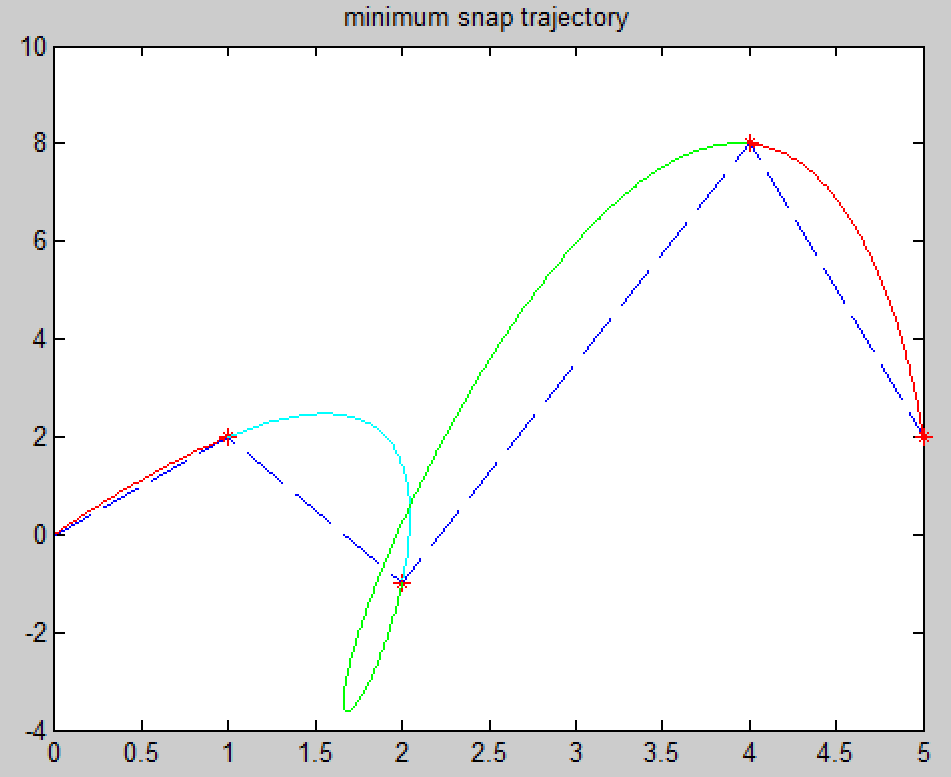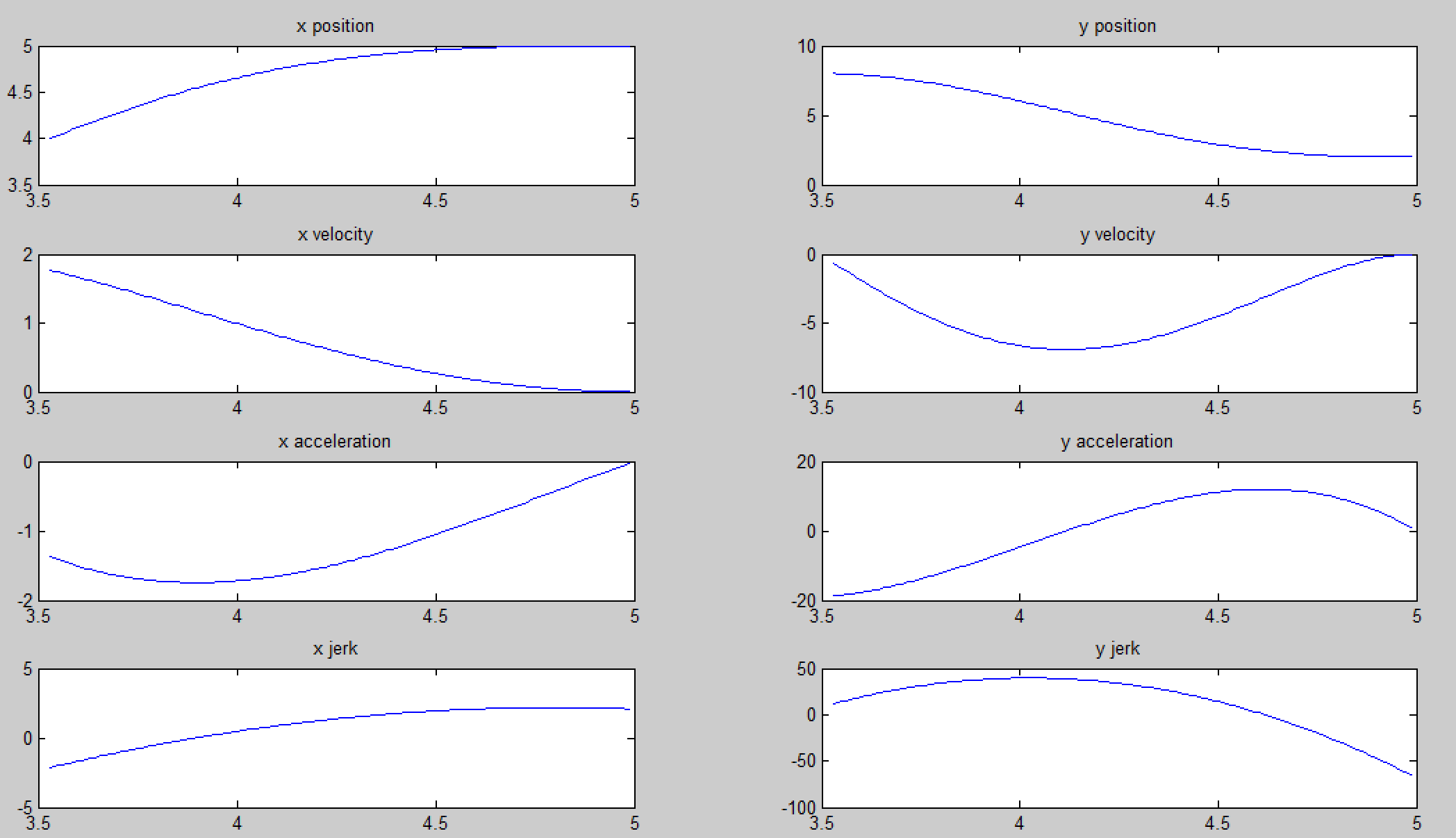5. 轨迹怎么用？（轨迹跟踪）

至此，我们已经求得了轨迹（很多段高阶多项式的参数），但怎么用来控制机器人运动呢？轨迹跟踪是：根据轨迹和机器人当前状态（当前位置、速度、加速度），输出机器人控制指令（速度、加速度、角速度等），控制机器人沿着轨迹运动。有很多种跟踪方法

最简单的跟踪方法是位置控制：计算轨迹上离当前位置最近的点，以最近点为期望位置做位置控制，即v=kp(pnearest−pcur)$v=k_p(p_{nearest}-p_{cur})$
Minimum Snap中的前馈控制：计算轨迹上离最近点的（位置pe$p_e$、速度ve$v_e$、加速度ae$a_e$），
速度指令：v加速度前馈：a=ve=ae+kp(pe−pcur)+kd(ve−vcur)\begin{equation}
\begin{aligned}
速度指令：v &= v_e\\
加速度前馈：a&=a_e+k_p(p_e-p_{cur})+k_d(v_e-v_{cur})
\end{aligned}
\end{equation}

6. 小结

轨迹规划问题通常建模成一个带约束的二次规划（QP）问题来求解，优化函数可以是snap、jerk、acceleration及它们的组合或其他任何能够formulate成pTQp$p^TQp$形式的函数，约束包括等式约束和不等式约束。
轨迹规划中默认时间t已知，通常根据期望速度和总路程计算一个总时间T，再按照匀速运动和梯形速度曲线分配到每段polynomial上。
上面例子中规划出的轨迹并不是很好，有以下问题：
a) 轨迹与路径相差有点大，而且在第三个waypoint处会有打结的现象；
b) y轴的加速度非常大（接近20m/s2$20m/s^2$），超过了机器人的最大加速度。实际轨迹需要进行feasibility check（可行性检测），确保满足工程可行性，比如最大速度、最大角速度限制等。
这两个问题的根本原因在于时间给的不合理，时间分配是轨迹规划中比较蛋疼的问题，给的时间太小，速度、加速度自然就很大，两段时间分配不当就会生成打结的轨迹。下一节，专门讨论时间分配问题。

参考文献

Richter C, Bry A, Roy N. Polynomial trajectory planning for aggressive quadrotor flight in dense indoor environments[M]//Robotics Research. Springer International Publishing, 2016: 649-666.
Vijay Kumar的一系列论文：Mellinger D, Kumar V. Minimum snap trajectory generation and control for quadrotors[C]//Robotics and Automation (ICRA), 2011 IEEE International Conference on. IEEE, 2011: 2520-2525.

展开全文• 轨迹规划是什么？ 在机器人导航过程中，如何控制机器人从A点移动到B点，通常称之为运动规划。运动规划一般又分为两步： 1、路径规划：在地图（栅格地图、四\八叉树、RRT地图等）中搜索一条从A点到B点的路径，由一...
• 轨迹规划定义 轨迹规划（Trajectory Planning）包括两个方面：对于移动机器人（mobile robot）偏向于指移动的路径轨迹规划（path planning），如机器人是在有地图条件或是没有地图的条件下，按什么样的路径轨迹来...
• 冗余机械臂matlab robotics 建模以及轨迹规划，修改参数方便快捷
• 关节空间的轨迹规划和笛卡尔空间的轨迹规划联系与区别 首先要有一个前提认识，那就是无论是关节空间的规划还是笛卡尔空间的规划，实现的目的只有一个:就是从笛卡尔空间坐标下的A点（x1,y1,z1,Yaw1，Pitch1，Roll1）...
• 我在上一篇博文07轨迹规划仿真中实现PTP的轨迹规划，并且把圆弧轨迹规划的坑挖好了，但是我一直很难受的地方就是终端的轨迹显示，我之前解释了我实习UR10moveit控制的方式，是使用官方panda的教程进行修改，但是...c++
• 在线轨迹规划 多项式在线轨迹规划 梯形在线轨迹规划 双S形在线轨迹规划 非线性实时轨迹滤波 多点轨迹（Multi-point) 三次样条曲线（cubic spline） 贝赛尔曲线（Bezier Curve） B样条曲线（BSpline） 时间...c++ pybind
• 轨迹规划 路径规划的目标是使路径与障碍物的距离尽量远同时路径的长度尽量短； 轨迹规划的目的主要是机器人关节空间移动中使得机器人的运行时间尽可能短，或者能量尽可能小。 轨迹规划在路径规划的基础上加入...
• 点到点的轨迹规划，加速度限制轨迹通过平均滤波器来产生Jerk 限制规制。 未解决任意初始加速度轨迹生成问题。matlab
• ## 机器人轨迹规划

千次阅读 2017-09-27 14:53:08
轨迹规划的目的生成运动控制系统的参考输入以确保机械手完成规划的轨迹路径规划的要求关节空间轨迹点对点运动插补方法 1、轨迹规划的目的：生成运动控制系统的参考输入，以确保机械手完成规划的轨迹。 ...
• 多项式轨迹规划，是对己上传的两个stm32实现工程的解释
• 该资源提供简单的RRT轨迹规划算法程序，里面附带五张地图，可以直接运行，方便初学者早早入门，希望对广大下载者有帮助！！！！！
• ## 运动规划/路径规划/轨迹规划

万次阅读 多人点赞 2017-01-07 16:01:27
引言查阅互联网资料与相关文献，略作总结，以期完善：运动规划、路径规划、轨迹规划的联系与区别？ motion planning path planning trajectory planningpath
• 但是我后来顿悟了，我是为了了解笛卡尔空间轨迹规划过程中的插值原理，尤其是四元数用于姿态的插值！我最终探讨的问题是： 如何实验笛卡尔空间下直线和圆弧的轨迹规划 并且在关节空间保证速度、加速度、加加速度的...
• 在线轨迹规划 多项式在线轨迹规划 梯形在线轨迹规划 双S形在线轨迹规划 非线性实时轨迹滤波 多点轨迹（Multi-point) 三次样条曲线（cubic spline） 贝赛尔曲线（Bezier Curve） B样条曲线（BSpline） 时间...三次多项式插值 python 机器人学
• 我的大三的机器人控制原理课程设计及，利用机器人工具箱，进行六自由度的机械臂的直线轨迹规划和圆弧轨迹规划，完全可用，参数可改，有注释说明
• 轨迹规划是无人驾驶系统的核心模块之一。轨迹规划模块承接上游感知、预测、决策等模块，规划无人驾驶车一段时间内的具体的驾驶行为，以轨迹的形式输出给下游控制模块执行。轨迹规划算法的优劣直接决定了无人驾驶车的...
• ## Minimum Snap轨迹规划

千次阅读 2019-11-05 16:12:11
Minimum Snap轨迹规划详解（1）轨迹规划入门 https://blog.csdn.net/q597967420/article/details/76099491 Minimum Snap轨迹规划详解（2）corridor与时间分配 ... Minimum Snap轨迹规划详...
• ## 路径规划和轨迹规划区别和联系

万次阅读 多人点赞 2019-01-15 16:30:49
1. 运动规划/路径规划/轨迹规划的联系与区别 https://blog.csdn.net/wx545644217/article/details/54175035 一、基本概念 运动规划Motion Planning 路径规划Path Planning 轨迹规划Trajectory Planning 运动...ROS
• 机器人轨迹规划仿真结果，施加点驱动，1)将机器人末端参考点的轨迹曲线的参数方程作为点驱动的参数，若参数方程数目小于自由度数目，需要对多余的运动参数 进行限制。2)添加驱动并仿真后利用后处理得到各驱动关节的......